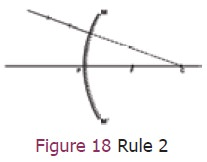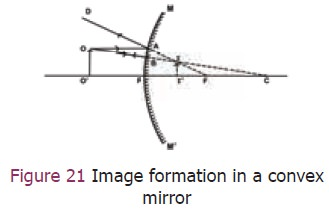Home | | Science 9th std | Convex Mirror

# Convex Mirror

We have studied the image formation by a concave mirror. Similarly, we can trace the path of light rays reflected by the convex mirrors using four ‘rules’.

Convex  Mirror

## 1. Rules for the construction of image formed by spherical mirrors

We have studied the image formation by a concave mirror. Similarly, we can trace the path of light rays reflected by the convex mirrors using four ‘rules’.

Rule 1: A ray of light which is parallel to the principal axis of a convex mirror appears to be coming from its principal focus, after reflection from the mirror (Figure 17).Rule 2: A ray of light going towards the centre of curvature is reflected back along the same path (Figure 18).Rule 3: A ray of light going towards the principal focus of a convex mirror becomes parallel to the principal axis after reflection (Figure 19).Rule 4: A ray of light which is incident at the pole of a convex mirror is reflected back making the same angle with the principal axis (Figure 20).## 2. Image formation in a convex  mirror

Any two rays can be chosen to draw the position of the image in a convex mirror (Figure 21).

1st ray: the ray that is parallel to the principal axis (rule 1) and

2nd ray : the ray that appears to pass through the centre of curvature (rule 2).

Note: All rays behind the convex mirror shall be shown with dotted lines.The ray OA parallel to the principal axis is reflected along AD. The ray OB retraces its path. The two reflected rays diverge but they appear to intersect at I when produced backwards. Thus II is the virtual image of the object OO. It is virtual, erect and smaller than the object.

### Sample Problem 3

A car is fitted with a convex mirror of focal length 20 cm. Another car is 6 m away from the first car.

a)     Find the position of the second car as seen in the mirror of the first

b)    What is the size of the image if the second car is 2 m broad and 1.6 m high?

Focal length     = 20 cm (convex mirror)

Object distance = -6m

= - 600 cm

Image distance v =?

Calculation for position of image using mirror equationb) Size of the image## 3. Uses of convex  mirror

Convex mirrors are used as rear-view mirrors in vehicles. It always forms a virtual, erect, small-sized image of the object. As the vehicles approach the driver from behind the size of the image increases. When the vehicles are moving away from the driver, then image size decreases. A convex mirror provides a much wider field of view* compared to plane mirror.### More to know by observation

1)    Have you ever seen the dish antenna used at your home? What is the shape of the antenna? Is it convex or concave? Why?

2)    Look around your environment. Observe all the spherical objects (having reflecting surfaces) and record your observation (for example soap bubble).

### Crossword puzzle#### Across

3. Kind of image formed when rays from the mirror converge.

4. Rays from an object at infinity.

6. Converging mirror.

9. Line perpendicular to the surface at the point of incidence.

11. Diameter of circular rim of spherical mirror.

#### Down

1. Reflection of light into many directions by rough objects.

2. The turning back of light at the shining surface of substance.

5. _______ of reflection: angle of incidence (i) = angle of reflection (r).

7. Centre of curvature is on the side opposite to the reflecting surface of mirror.

8. Image of an object in a plane mirror.

10. _______ of reflection angle between the reflected ray and the normal at thepoint of contact.

12. Nature of image formed by convex mirror.

Study Material, Lecturing Notes, Assignment, Reference, Wiki description explanation, brief detail
9th Science : Light : Convex Mirror |